#### The quantifier-elimination problem

Another important problem was exemplified in (6.12). Consider the set of all Tarski sentences of the form (6.9), which may or may not have free variables. Can an algorithm be developed that takes a Tarski sentence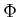and produces an equivalent quantifier-free formula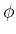? Let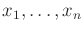denote the free variables. To be equivalent, both must take on the same true values over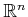, which is the set of all assignments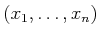for the free variables.

Given a Tarski sentence,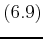, the quantifier-elimination problem is to find a quantifier-free formulasuch that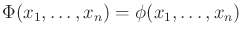(6.14)

for all. This is equivalent to constructing a semi-algebraic model becausecan always be expressed in the form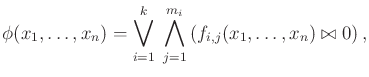(6.15)

in which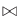may be either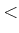,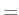, or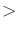. This appears to be the same (3.6), except that (6.15) uses the relations,, andto allow open and closed semi-algebraic sets, whereas (3.6) only used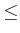to construct closed semi-algebraic sets for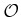and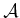.

Once again, the problem is defined on, which is uncountably infinite, but an algorithm must work with a finite representation. This will be achieved by the cell decomposition technique presented in Section 6.4.2.

Steven M LaValle 2020-08-14